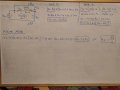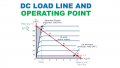# Finally I figured it out on how to control saturation and active modes!

#### babaliaris

Joined Nov 19, 2019
115
Just to be sure, I want you to review my conclusion and tell me if I'm correct. If I have a transistor with 3 resistors (one for every pin of the transistor) Rb, Rc and Re (base, collector and emitter) then I can conclude the following:

1) Re does not affect the mode.
2) If $$R_{b} > bR_{c}$$ then the transistor will be on active mode, else on saturation.
3) I noticed by experimenting, that if I increase Ve (on active mode), then Vb and Vc are also being increased.

This is how I concluded that $$R_{b} > bR_{c}$$:I tested this theory and it seems to work.
Of course on different setups, I might need to do this work again to figure it out.

#### Jony130

Joined Feb 17, 2009
5,351
•babaliaris

#### Jony130

Joined Feb 17, 2009
5,351
3) I noticed by experimenting, that if I increase Ve (on active mode), then Vb and Vc are also being increased.
When BJT is in the active mode and as Vb and Ve increases for a constant Rc the collector voltage (Vc) will decreases.

•babaliaris

#### babaliaris

Joined Nov 19, 2019
115
Yes, this is true in general. But I do not like your loop A. Normally we do loop B as you Vin = Ib*Rb + Vbe + Ie*Re
And Loop C:
Vin = Ic*Rc + Vce + Ie*Re.

Vc = Vin - Ic*Rc
Ve = Ie*Re

And for a BJT's the Vb - Ve = Vbe = 0.6V...0.8V therefore Vb = Ve+Vbe ≈ Ve+0.6V
Thank you for the tip! It was actually your help from my previous post that made me understand and conclude this. Finally, I'm ready to be able to figure out how to synthesize some simple transistor circuits and understand how they work!

Now I think I must learn Thevenin as good as possible because If I'm right, the purpose of this theorem is to model the linear part of the transistor circuit to just one voltage source in series with a resistor and this will make it so much simpler to calculate the voltages of the transistor, even if I connect different types of transistors, right?

#### Jony130

Joined Feb 17, 2009
5,351
We use the Thevenin's theorem to simplify the circuit and eliminate this voltage divider at the base.We can replace the voltage divider (this gray rectangle) with his Thevenin's equivalent circuit

$V_{TH} = V_{CC} \times \frac{R_2}{R_1+R_2}$

$R_{TH} = R_1||R_2 =\frac{R_1 \times R_2}{R_1+R_2}$

As you can see this Thevenin is very simple in this case.

#### MrChips

Joined Oct 2, 2009
26,490
Your approach is wrong. You are fixated on the choice of resistors. It is true that resistors will determine the operating current and voltage. You need to focus on the desired operating current and voltage and not on the value of the resistor.Begin by selecting a supply voltage Vcc within the operating voltages of the transistor and desired circuit application.
For example, in the graph above, Vcc is chosen to be about 6.5V.

Next you choose the max Ic (or the load resistance = Vcc / Icmax or Icmax = Vcc/RLOAD).
In the example, Icmax = 70mA.
RLOAD = Vcc / Icmax = 6.5V / 70mA ≅ 100Ω

Look carefully at the diagram above. The red line is called the load line.
For any transistor bias you choose, the transistor operating current and voltage will fall on some point along the load line.
If you choose point A, the transistor is saturated.
At point B, the transistor is cut-off.
To operate the transistor in the linear region, choose point Q, called the Q-point, which is about half-way along the load line.

•babaliaris

#### Audioguru again

Joined Oct 21, 2019
4,796
The beta of a transistor is a range of numbers. If you want every passing transistor to work in a circuit then the circuit should be designed with "worst case" low and high beta that are printed on the datasheet. The graphs on a datasheet are almost always showing "typical" beta which you cannot buy, you get whatever beta is available.

#### OBW0549

Joined Mar 2, 2015
3,566
Now I think I must learn Thevenin as good as possible because If I'm right, the purpose of this theorem is to model the linear part of the transistor circuit to just one voltage source in series with a resistor...
Well, that's not the "purpose" of the theorem, which is true regardless of whether you're talking about transistor circuits or something else.

Reducing a portion of a circuit to its Thevenin equivalent (or, alternatively, to its Norton equivalent) is a method for circuit simplification for analysis purposes; when applied appropriately it can speed up the task of analyzing a circuit and/or make the circuit easier to understand.

Being able to reduce a circuit to its Thevenin equivalent is a basic circuit design skill. If you expect to progress very far as a designer you need to master that skill to the point where you can do it almost automatically without having to think too hard about it.

•babaliaris